Suppose that net exports, NXt, are determined by the following expression:

NXt/Ȳt = αNX−βNX(rt−rt) (6),

where t is potential GDP and rt−rt is the difference between the domestic and the foreign real interest rate.

a. Explain the intuition behind expression (6).

b. Modify the IS curve below so that (6) is taken into account.

t= α − β (rt−r̃)

It specifies a negative relationship between short-run output, Ỹt, and the real interest rate, rt

c. Explain how a decrease in the domestic real interest rate affects short-run output in this economy.

Similar Homework Help Questions
• ### Hi, I need answer for this question below.BR//Hassan suppose that net exports, NXt , are determined...

Hi, I need answer for this question below.BR//Hassan suppose that net exports, NXt , are determined by the following expression: NXt Y¯ t = αNX − βNX(rt − r ∗ t ), where Y¯ t is potential GDP and rt − r ∗ t is the difference between the domestic and the foreign real interest rate. a. Explain the intution behind expression . b. Modify the IS curve . c. Explain how an increase in the domestic real interest rate...

• ### Please I need today answer for This question and it is very important and I need...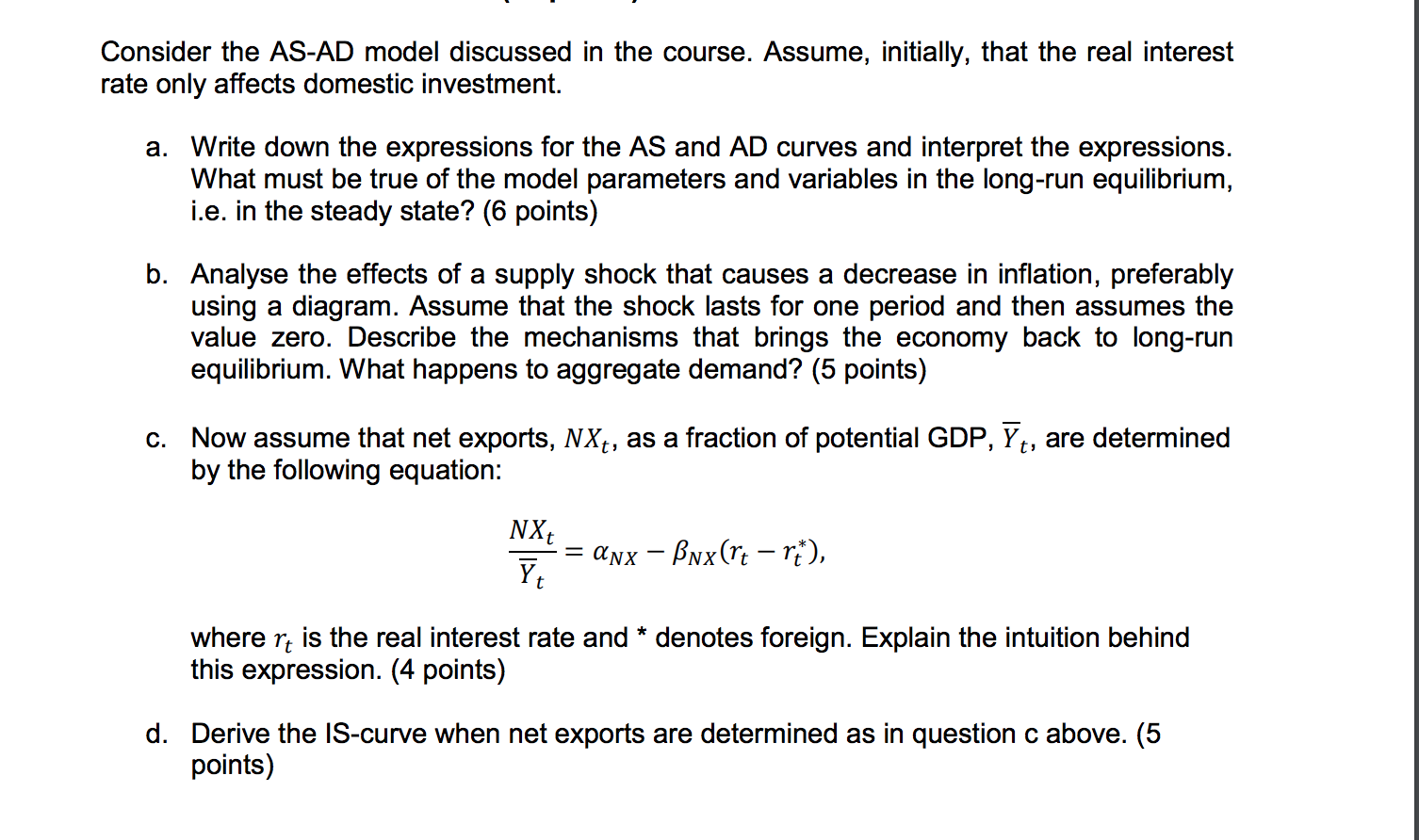Please I need today answer for This question and it is very important and I need solution for this issue with all the details , and help me with all the details.Please write your answer to me by typing, not by handwriting, so that I can read and understand your answer clearly.BR/Hassan. Consider the AS-AD model discussed in the course. Assume, initially, that the real interest rate only affects domestic investment. a. Write down the expressions for the AS and...

• ### Hi, Please find some solution for this question below. I need your answer for this question...

Hi, Please find some solution for this question below. I need your answer for this question very  quickly.Br/H Consider the AS-AD model . Assume, initially, that the real interest rate only affects domestic investment. a. Write down the expressions for the AS and AD curves and interpret the expressions. What must be true of the model parameters and variables in the long-run equilibrium, i.e. in the steady state? b. Analyse the effects of a supply shock that causes a decrease in...

• ### PLEASE provide an answer for Q2 and Q3 and their subquestions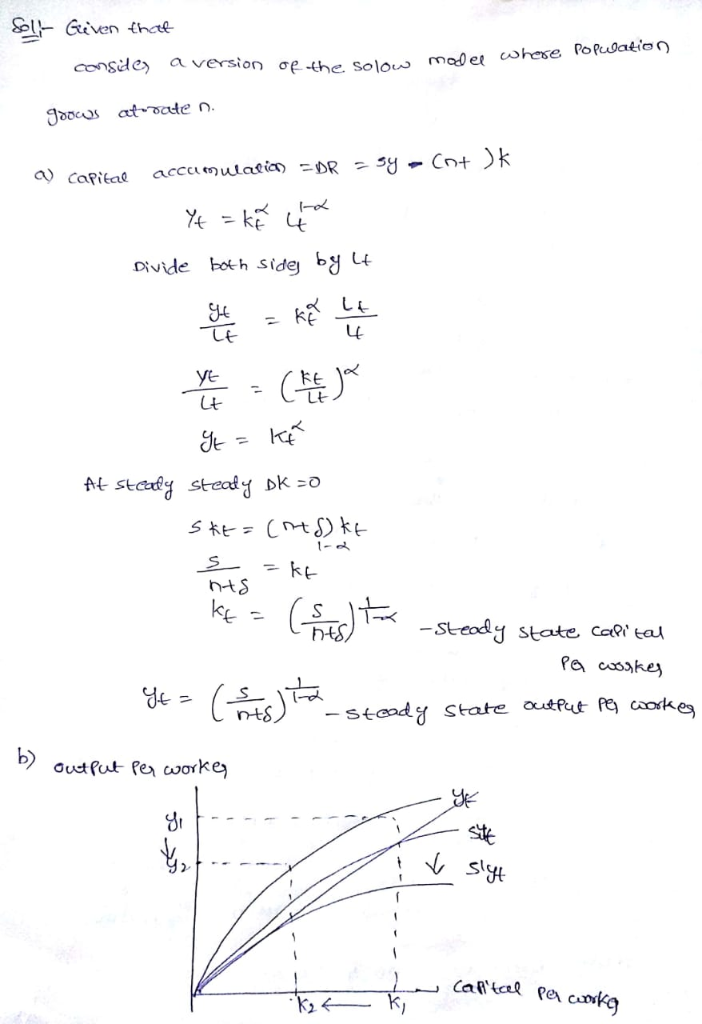Q2)Consider two imaginary countries, indexed A and B. Each economy can be characterised by the model above, but the population is constant in both economies. In the steady state, GDP per worker in country A is 1.44 times that of country B and the ratio of physical investment to output is 0.3 in country A and 0.25 in country B. The rate of depreciation is the same in both countries. What must α be in order for the model to...

• ### Describe the effects of contractionary monetary policy by the domestic central bank on output, the real...

Describe the effects of contractionary monetary policy by the domestic central bank on output, the real interest rate, and net exports in both the domestic and foreign country, using a Keynesian model in the short run. What happens in the long run? (Word Limit: 100 words)

• ### Question 9 The Taylor rule expresses the federal funds rate as the weighted average of: A....

Question 9 The Taylor rule expresses the federal funds rate as the weighted average of: A. inflation and short-run output B. the unemployment rate and inflation C. he misery index, the money growth rate, and the mortgage rate D. the CPI and real GDP Question 10 The real exchange rate measures the: A. number of foreign goods one unit of domestic currency can buy B. number of foreign goods required to purchase a single unit of a domestic good C....

• ### Problem 1: Consider the following GDP equation, consumption function, exports function. imports function and investment function...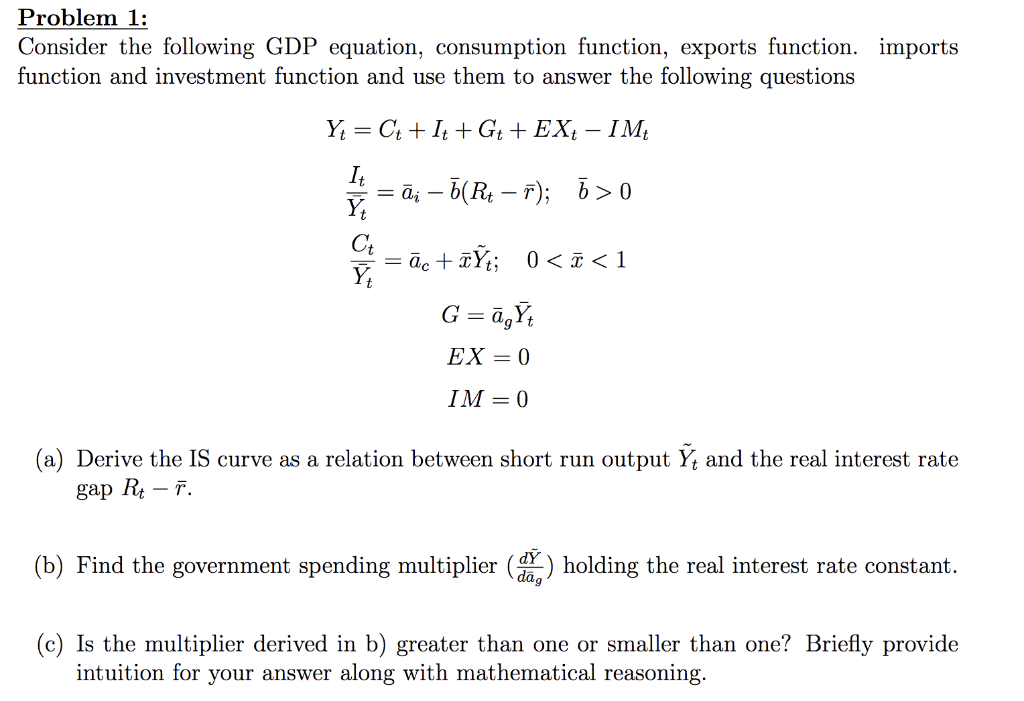Problem 1: Consider the following GDP equation, consumption function, exports function. imports function and investment function and use them to answer the following questions Y; = C4 + 1++G+ + EX, – IM le = ā; – Õ(R4 – 7); 7 > 0 = āc + TÝ; 0<ī<1 G=ā, EX = 0 IM = 0 (a) Derive the IS curve as a relation between short run output Yt and the real interest rate gap Rt – Ť. (b) Find the...

• ### Question 3 (25 points) - Chapter 6 Suppose the small open economy Iceland has perfect financial...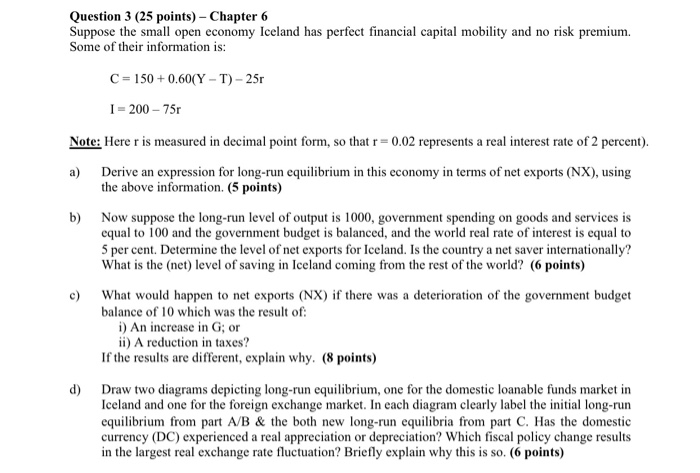Question 3 (25 points) - Chapter 6 Suppose the small open economy Iceland has perfect financial capital mobility and no risk premium. Some of their information is: C = 150 +0.60(Y - T) - 25 I=200 - 750 Note: Herer is measured in decimal point form, so that r = 0.02 represents a real interest rate of 2 percent). a) Derive an expression for long-run equilibrium in this economy in terms of net exports (NX), using the above information. (5...

• ### 2. Consider the following short-ru model of an open economy: Y C+I+G+NX = 50 IM = -EY The domesti...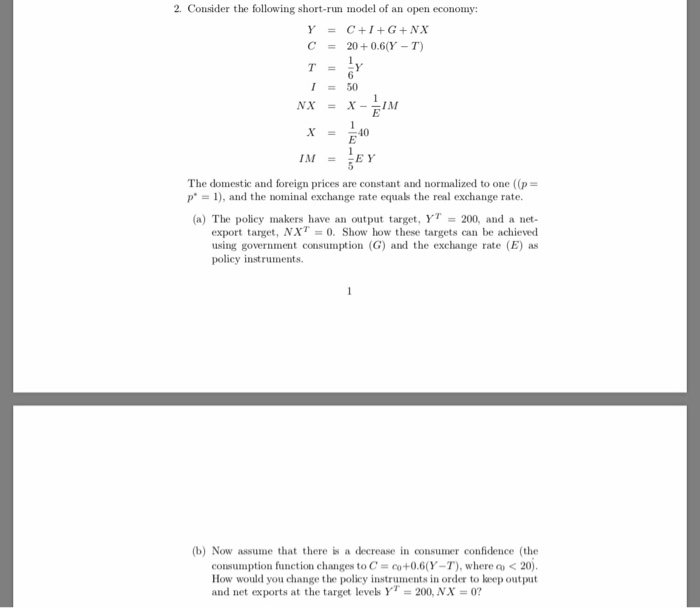2. Consider the following short-ru model of an open economy: Y C+I+G+NX = 50 IM = -EY The domestic and foreign prices are constant and normalized to one ((p p" 1), and the nominal exchange rate equals the real exchange rate. (a) The policy makers have an output target, YT 200, and a net- export target, NXT = 0' Show how these targets can be achieved using government consumption (G) and the exchange rate (E) as policy instruments (b) Now...

• ### Macroeconomics

Suppose political stability in the US as seen from abroad deteriorates and US assetsbecome less attractive to foreign investors. Assume that the US is a large openeconomy and that neither Congress nor the Federal Reserve Board takes action tocounter the speculative capital outflow.(1) Using the Mundell-Fleming model for a large open economy, state and showgraphically what would happen in the short run to each of the following:A) the US net capital outflow curveB) the IS and LM curvesC) the domestic...

Free Homework App